#Boost C++ Libraries

...one of the most highly regarded and expertly designed C++ library projects in the world.

## Struct template peaks_over_threshold_impl

boost::accumulators::impl::peaks_over_threshold_impl — Peaks over Threshold Method for Quantile and Tail Mean Estimation.

## Synopsis

```// In header: <boost/accumulators/statistics/peaks_over_threshold.hpp>

template<typename Sample, typename LeftRight>
struct peaks_over_threshold_impl {
// types
typedef numeric::functional::average< Sample, std::size_t >::result_type float_type;
typedef boost::tuple< float_type, float_type, float_type >               result_type;
typedef mpl::int_< is_same< LeftRight, left >::value?-1:1 >              sign;

// construct/copy/destruct
template<typename Args> peaks_over_threshold_impl(Args const &);

// public member functions
template<typename Args> void operator()(Args const &) ;
template<typename Args> result_type result(Args const &) const;
};```

## Description

According to the theorem of Pickands-Balkema-de Haan, the distribution function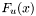of the excessesover some sufficiently high threshold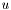of a distribution functionmay be approximated by a generalized Pareto distribution

Equation 1.3.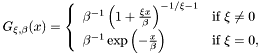with suitable parametersandthat can be estimated, e.g., with the method of moments, cf. Hosking and Wallis (1987),

Equation 1.4.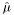andbeing the empirical mean and variance of the samples over the threshold. Equivalently, the distribution functionof the exceedancescan be approximated by. Since forthe distribution functioncan be written as

Equation 1.5.and the probabilitycan be approximated by the empirical distribution functionevaluated at, an estimator ofis given by

Equation 1.6.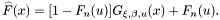It can be shown thatis a generalized Pareto distributionwithand. By inverting, one obtains an estimator for the-quantile,

Equation 1.7.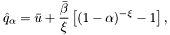and similarly an estimator for the (coherent) tail mean,

Equation 1.8.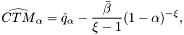cf. McNeil and Frey (2000).

Note that in case extreme values of the left tail are fitted, the distribution is mirrored with respect to theaxis such that the left tail can be treated as a right tail. The computed fit parameters thus define the Pareto distribution that fits the mirrored left tail. When quantities like a quantile or a tail mean are computed using the fit parameters obtained from the mirrored data, the result is mirrored back, yielding the correct result.

For further details, see

J. R. M. Hosking and J. R. Wallis, Parameter and quantile estimation for the generalized Pareto distribution, Technometrics, Volume 29, 1987, p. 339-349

A. J. McNeil and R. Frey, Estimation of Tail-Related Risk Measures for Heteroscedastic Financial Time Series: an Extreme Value Approach, Journal of Empirical Finance, Volume 7, 2000, p. 271-300

### `peaks_over_threshold_impl` public construct/copy/destruct

1. `template<typename Args> peaks_over_threshold_impl(Args const & args);`

### `peaks_over_threshold_impl` public member functions

1. `template<typename Args> void operator()(Args const & args) ;`
2. `template<typename Args> result_type result(Args const & args) const;`